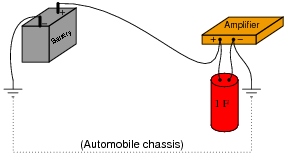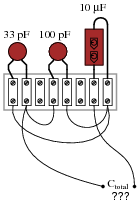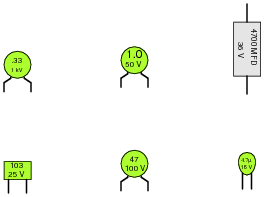# Capacitors

## DC Electric Circuits

• #### Question 1

Identify some of the different types of capacitors, and their characteristics.

• #### Question 2

How are electrolytic capacitors constructed, and what is particularly noteworthy about their use?

• #### Question 3

A capacitor has a label on it saying, “100 WVDC”. What does this label mean? What is the consequence of exceeding this rating?

• #### Question 4

Capacitors may pose an electric shock hazard, even in unpowered circuits. Explain why.

• #### Question 5

Very large capacitors (typically in excess of 1 Farad!) are often used in the DC power wiring of high-power audio amplifier systems installed in automobiles. The capacitors are connected in parallel with the amplifier’s DC power terminals, as close to the amplifier as possible, like this:What is the purpose of having a capacitor connected in parallel with the amplifier’s power terminals? What benefit does this give to the audio system, overall?

• #### Question 6

A 10 μF capacitor is charged to a voltage of 20 volts. How many coulombs of electric charge are stored in this capacitor?

• #### Question 7

A 470 μF capacitor is subjected to an applied voltage that changes at a rate of 200 volts per second. How much current will there be “through” this capacitor?

Explain why I placed quotation marks around the word “through” in the previous sentence. Why can’t this word be used in its fullest sense when describing electric current in a capacitor circuit?

• #### Question 8

Two 470 μF capacitors connected in series are subjected to a total applied voltage that changes at a rate of 200 volts per second. How much current will there be “through” these capacitors? Hint: the total voltage is divided evenly between the two capacitors.

Now suppose that two 470 μF capacitors connected in parallel are subjected to the same total applied voltage (changing at a rate of 200 volts per second). How much total current will there be “through” these capacitors?

• #### Question 9

Suppose two 33 μF capacitors are connected in series with each other. What will their combined capacitance be, in Farads? Explain your answer.

• #### Question 10

Calculate the total capacitance in this collection of capacitors, as measured between the two wires:• #### Question 11

Identify the following capacitor values and styles:• #### Question 12

When checked with an ohmmeter, how should a properly functioning capacitor respond?

• #### Question 13

Find one or two real capacitors and bring them with you to class for discussion. Identify as much information as you can about your capacitors prior to discussion:

Capacitance (ideal)
Capacitance (actual)
Voltage rating
Type (mica, mylar, electrolytic, etc.)
• #### Question 14

An important parameter in capacitor performance is ESR. Define ESR, and explain what causes it.

• #### Question 15

Capacitors often have letter codes following the three-digit number codes. For example, here are some typical capacitor codes, complete with letters:

473K
102J
224M
331F

Determine the meaning of letters used on capacitor labels, what the respective numeric values are for all the available letters, and then finally what these four specific number/letter codes mean (shown above).